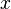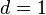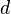# Thin-walled tank/Introduction

Thin-walled tank tutorial

### The Problem

You would like to compute the weight of a thin-walled cylindrical tank made of mild steel as shown in Fig. 1. The diameter of the tank is 20 cm, the height 50 cm, the wall thickness 0.15 cm, and the density of mild steel 7.85 g/cc.

### Analysis

Being thin walled, we will approximate the volume of metal to be the area of the tank walls times the wall thickness. The following formulas hold:

```area of the cylindrical wall = π*diameter*height
area of two flat end pieces = 2 end pieces*π*(diameter)^2/4
```

where the volume of metal is then the wall thickness times the sum of these two areas. Finally the mass of metal would be the metal density times this volume.

## Writing our first model

We construct the following ASCEND model for this problem.

```(* this is file twt0010.a4c *)
REQUIRE "system.a4l";

MODEL thin_walled_tank;
(* variables *)
D,
H,
wall_thickness,
metal_density,

end_area,
side_area,
wall_vol,
metal_mass      IS_A solver_var;

(* specifications *)
D              = 20.0;
H              = 50.0;

wall_thickness = 0.15;
metal_density  = 7.85;

(* equations *)

end_area = 3.1416*D^2/4;
side_area = 3.1416*D*H;

(side_area+2*end_area)*wall_thickness = wall_vol;
metal_mass = metal_density*wall_vol;

END thin_walled_tank;
```

This MODEL is quite straight forward to write. ASCEND uses strong typing; it requires one to declare explicitly the type of each variable using IS_A statements. Any variable whose value it is expected to compute must be of at least type "solver_var," the type we have used here. (Note that we insert a first statement to "require" that a file called "system.a4l" loads first as it contains the definition for the type "solver_var.")

We have written the entire model in the form of equations, including the equations we used to specify D, H, wall_thickness and metal_density. ASCEND will organize how to solve these equations irrespective of how we have written them. Note that we deliberately entered the third equation in a nonintuitive order to emphasize that ASCEND only needs the equations in the form that the expression on the right hand side equals the expression on the left hand side.

## Solving our first model

Start up the tcl interface for ASCEND. Then load this model and compile it. You will see, when you export this model to the Browser, that ASCEND has set all variables to a default of 0.5 and the initial equation residuals (tank_1 to _4) to a very large number.

Export this model to the solver and solve it. The residuals should all become essentially zero while the variables will have their correct computed values - in particular, metal_mass will be 4439 g.

You may now play with different values for the fixed variables by editing new values into their respective equations in the model code, reloading and recompiling. While playing, try to guess a value for D that will give a metal_mass of 5000 g while keeping all the remaining specified variables unchanged (D will be just over 22 cm). Seriously, do try this last exercise. It will help you to appreciate the next section.

### Solving with the pygtk interface

Using exactly the same model, start the PyGTK interface. Next load the model and directly solve it. The ease with which you just did these steps is an advantage of this interface. You should examine the various sections of the interactive window when using this interface to gain some familiarity with it.

### More simple examples

#### 1

Solve

```x*cos(x) = 1
```

for$x$.

Try coding this yourself using the above thin walled tank example as a guide for correct ASCEND syntax. Then compare what you wrote to the code we give here.

#### 2

Solve

```x + y + z = 6*d
x - y + 2*z = 5
5*x + 2*y - 3*z = 0
```

for$d = 1$.

Again, try coding and solving this problem yourself. Note that you are solving a set of three linear equations here, a task that is straight forward for ASCEND. Our code is here. Change the value for$d$ and resolve.

## Solving for D when the metal mass is fixed

Suppose you would now like to compute a value for the tank diameter for a case where the metal_mass is 5000 g rather than the 4439 g ASCEND just computed for it. If you set up this model in Excel, you could do this type of reverse computation by using the Excel solver tool. This tool mimics what you just did in the previous section: it repeatedly reguesses D until it can make metal_mass equal to 5000 g. Of course it uses great numerical techniques to do the reguessing so it will be quick to do it.

A simple way to do this new computation in ASCEND is to remove the statement specifying D and replace it with one specifying metal_mass.

```metal_mass = 5000;
```

Then load, compile and solve as before using either interface (for the fun of it, do this solving with one interface and then the other so you become more comfortable with both). Note, you do NOT have to reorganize the equations. That ASCEND can discover a way to solve, even if it must solve some or all of the equations simultaneously, is what makes using ASCEND very different from using Excel. Try it. You should get a new diameter of 22.13 cm.

## Using fixed flags to choose which variables we wish to specify

Modifying the model and then reloading, compiling and solving is a bit tedious when we want to change values for the specified variables or when we want to change which of the variables we wish to specify. It is especially tedious if compiling is not as instantaneous as it seems to be for this small model. ASCEND provides a whole set of capabilities in model writing and in interactively browsing and solving to handle this problem.

We rewrite our model by supplying ONLY the model equations and not the specifications.

```(* this is file twt0020.a4c *)
REQUIRE "system.a4l";

MODEL thin_walled_tank;

(* variables *)
D,
H,
wall_thickness,
metal_density,

end_area,
side_area,
wall_vol,
metal_mass      IS_A solver_var;

(* equations *)
end_area = 3.1416*D^2/4;
side_area = 3.1416*D*H;

(side_area+2*end_area)*wall_thickness = wall_vol;
metal_mass = metal_density*wall_vol;

END thin_walled_tank;
```

Returning to the tcl interface for ASCEND, we load this modified model and compile it as before. Now when we attempt to solve, ASCEND complains that the model is underspecified. It is noticing that it has eight variables and only four equations to use to compute their values. It wants you to select some of the variable to "fix." Select in turn D, H, wall_thickness and metal_density. If you then solve the model, it will solve but it will use the default values of 0.5 for all these specified variables.

To set the values we really want for these four variables, we can return to the Browser window, RIGHT mouse pick the variable of interest and type in the desired value for it. Do this for all four of these variables (D=20.0, H=50.0, wall_thickness=0.15 and metal_density=7.85) and solve the model again.

Now LEFT mouse pick D. You will see that D has parts, one of which is a "fixed" flag that is currently set to TRUE. The same will be true for H, wall_thickness and metal_density. For the remaining variables, this flag will have the value FALSE. The solver will treat all the fixed variables (value TRUE) as the ones whose values are fixed when solving - i.e., they are treated as the specified variables.

Now RIGHT mouse pick this flag for D and set its value to FALSE. If you now attempt to solve, ASCEND will again complain that the model is underspecified and offer you a list of variables, one of which you need to fix. This time select metal_mass. In the Browser, reset the value for metal_mass to 5000, and solve the model.

Note you did not reload and then compile this model to make these changes. So you can pick any legitimate set of four variables to fix, and ASCEND will compute the other four. So why qualify this statement with the word "legitimate?" Suppose you picked to fix both D and end_area? There is an equation relating them. You can fix only one; the equation will determine the value for the other. Sending a model to the solver starts up a tool that checks if your set of four variables form a legitimate specification set. It will complain if it does not and guide you to modify which variables you selected to be fixed until the set is legitimate.

### Repeat above with the PyGTK interface

Restart the PyGTK interface and load this version of the model.

## Dimensionality and units in ASCEND

The previous computations required us to express all the variable values using a set of consistent units. If, for example, we want the height, H, to be 2 ft rather than 50 cm, we would have to convert manually 2 ft into 60.96 cm and use this converted value in the model. ASCEND is a lot smarter than this. It knows all about dimensionality and units. The variables D, H, and wall_thickness all have the dimensionality of distance and can be expressed in any units consistent with that dimensionality, such as in ft, in, cm, m, furlongs, or whatever. To unleash this capability in ASCEND, we have to tell ASCEND the type of each variable. The file atoms.a4l in the models directory lists a very large number the types. If we load it first, ASCEND will know all about these types, any of which we may then use in our model. Don't worry if a type you need is not there, you can create your own file defining the new types and load it right after loading atoms.a4l. And don't worry that you will have to search atoms.a4l to find a type you may need. There is a nice tool available within the TclTk interface (as yet an equivalent is not available in the PyGTK interface) that will list all types that one can express in the units of "g/cm^3," for example.

We rewrite the following code to reflect the variable types so we can then express variables along with their units and allow ASCEND to handle units conversions. The types we use here are all in atoms.a4l. We place a REQUIRE statement before the tank statements in our model file to cause ASCEND to load the atoms.a4l file first (which itself includes a REQUIRE "system.a4l"; statement).

```(* this is file twt0030.a4c *)
REQUIRE "atoms.a4l";

MODEL thin_walled_tank;

(* fixed variables *)

D,
H,
wall_thickness  IS_A distance;
metal_density   IS_A mass_density;

(* computed variables *)
end_area,
side_area       IS_A area;
wall_vol        IS_A volume;

metal_mass      IS_A mass;

(* specifications *)
D              = 20.0 {cm};

H              = 2.0 {ft};
wall_thickness = 0.15 {cm};

metal_density  = 7.85 {g/cm^3};

(* equations *)
end_area=3.1416*D^2/4;

side_area=3.1416*D*H;
wall_vol=(side_area+2*end_area)*wall_thickness;

metal_mass=metal_density*wall_vol;

END thin_walled_tank;
```

We have left the equations that set the specifications in this version of our model as we are only interested here in understanding a bit about dimensions and units in ASCEND. Note, we specified H to be 2 ft.

ASCEND converts all variables internally to be in the SI system of units before doing any computations with them. Thus if you could see into the actual storage location in the computer, you would find D stored as the real 0.02 (the value in meters), H as 0.06096 and so forth. When the variables are all in the SI system of units, these equations all work as written.

In both interactive interfaces, ASCEND can report the variable values back to you in any units you wish. If you wish to try out this feature in the TclTk interface, you need to use the Units Tool set. In the PyGTK interface, you can simply right mouse the variable and select to set its display units.

Continue with the next section, Adding methods to a model.